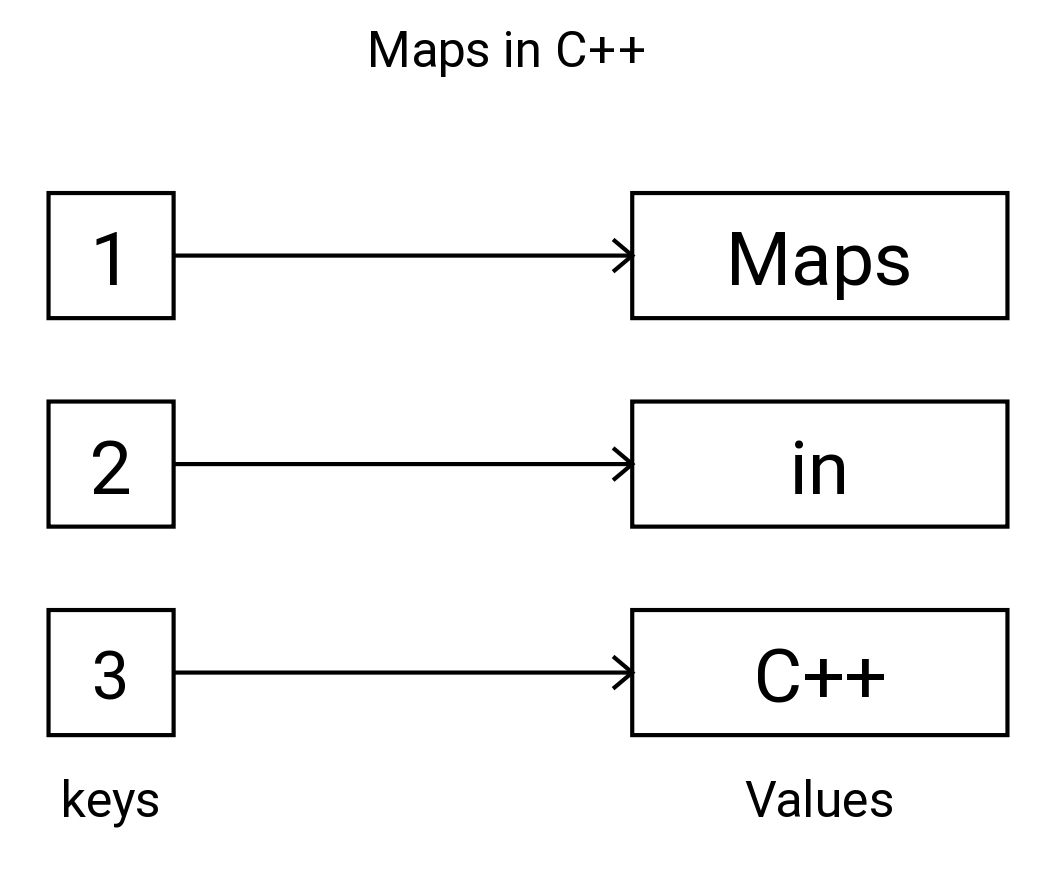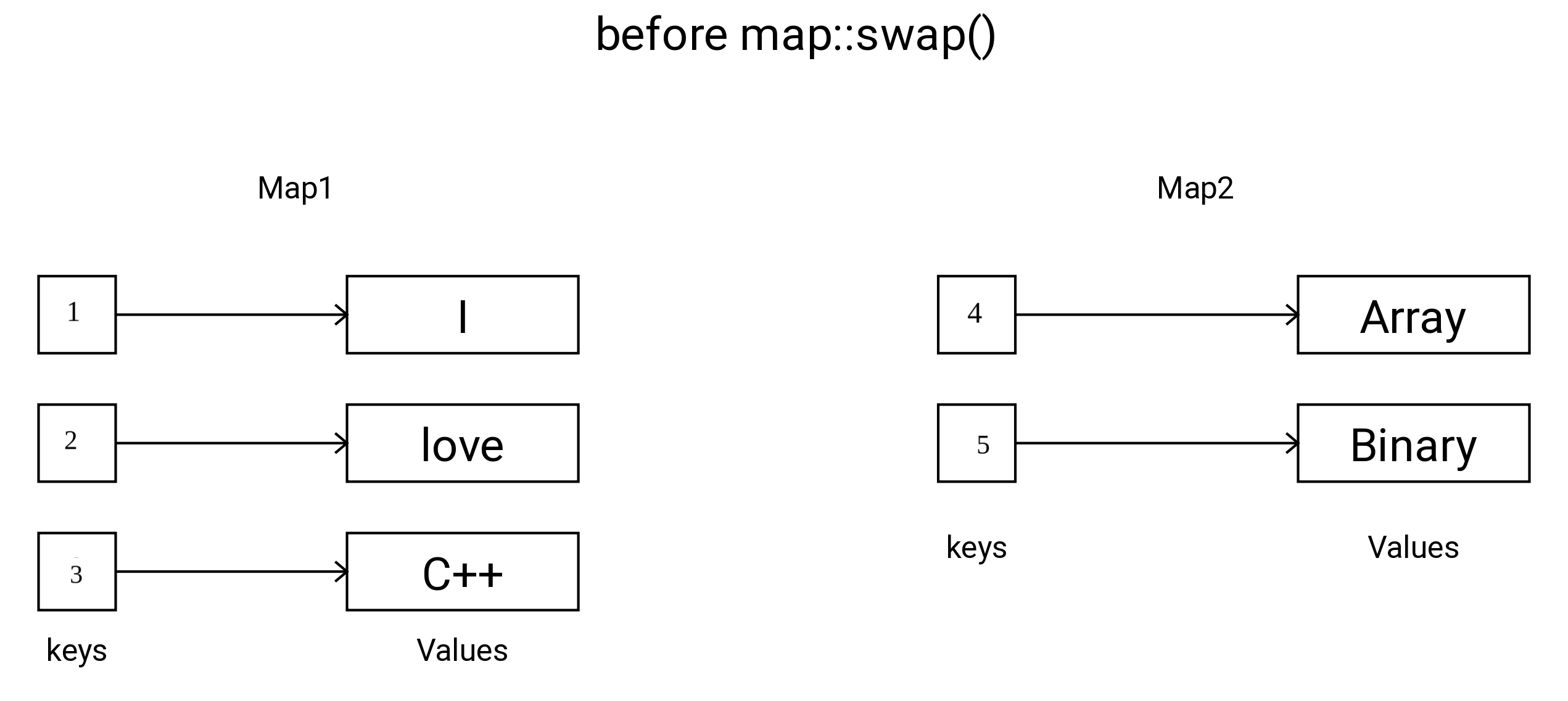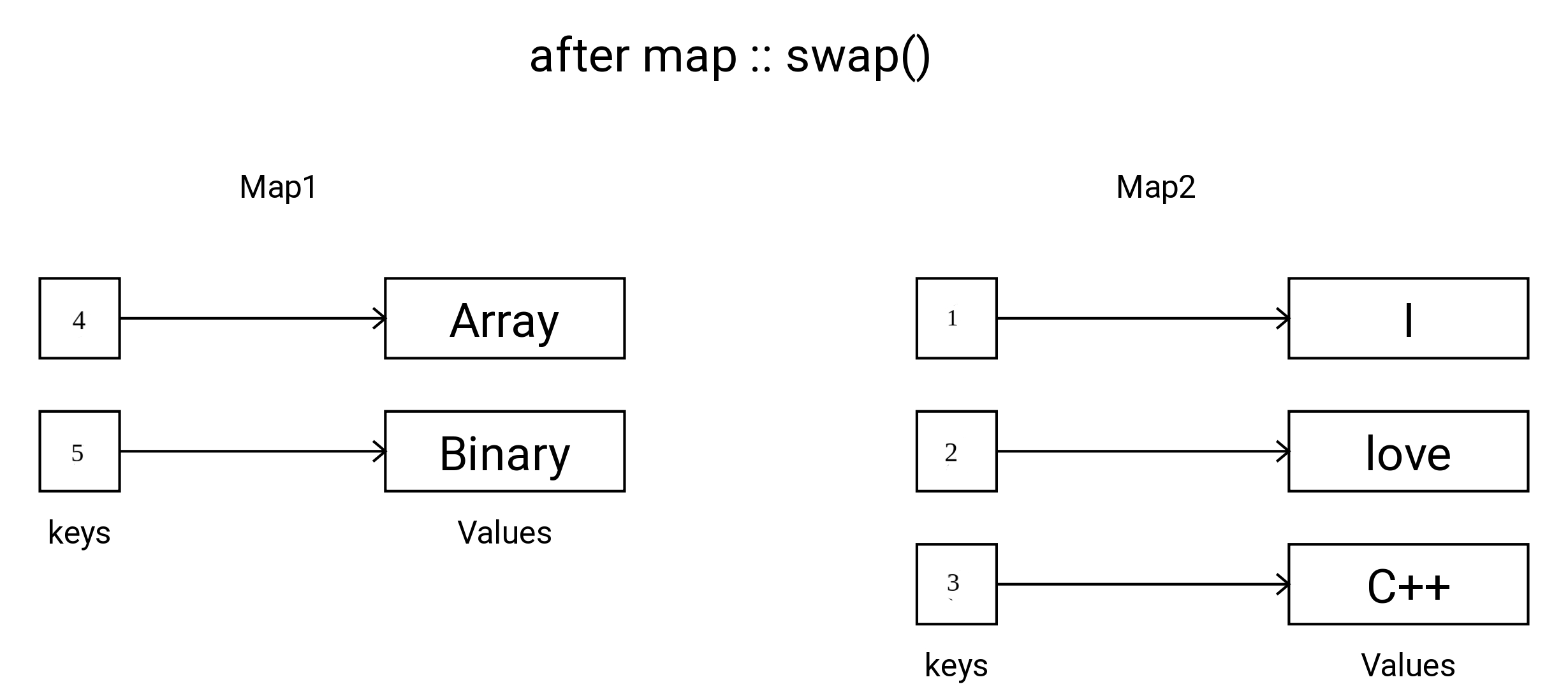×

Search anything:

# Map::swap() in C++

#### C++Get this book -> Problems on Array: For Interviews and Competitive Programming

Table of content:

1. Introduction to Maps
2. Detailed view of Map::swap()
3. Syntax to swap two Maps
4. Examples to swap two maps
5. Time and Space complexity
6. Application
7. Questions

# Introduction to Maps

In C++, a map is an associative container provided by the Standard Template Library (STL) that stores elements in a sorted manner, allowing for efficient searching. It has a time complexity of O(log n) for operations like insertion, deletion, and search.

A map stores elements in a mapped manner, where each element has a unique key associated with its value. The keys are sorted internally, enabling fast retrieval and traversal of elements based on their key values. This ordering property of maps makes them useful when elements need to be accessed in a sorted manner or when efficient key-based lookups are required.Here we talk about one of the function of Map, which is Map::swap()

# Detailed view of Map::swap()

The swap() function in C++ is used to interchange the elements of two variables. Similarly, the Map::swap() member function is used to swap the elements of two map objects.

The swap() function in C++ is a generic algorithm that exchanges the values of two objects, effectively swapping their contents. It can be used with various data types, including fundamental types and user-defined types, to quickly exchange their values.

In the case of maps, the Map::swap() member function specifically swaps the elements of two map objects. When called on a map instance, it exchanges its contents with another map, effectively swapping all key-value pairs between them. This operation is performed efficiently, as it involves a direct exchange of internal data structures rather than individual element-by-element swaps.

Using the swap() function or the Map::swap() member function can be useful when there is a need to efficiently exchange the contents of two maps, avoiding the need for manual element-by-element swapping or copying.Note: For swapping, Maps must be of same type, although sizes may differ.

# Syntax to swap two Maps

Maps can be swapped in C++ using two methods:

1. std::swap(Map1, Map2): This method uses the std::swap function to exchange the contents of Map1 and Map2. After the swap, Map1 will contain the elements that were originally in Map2, and Map2 will contain the elements that were originally in Map1.
2. Map1.swap(Map2): This method swaps the contents of Map1 and Map2. Similar to the first method, after the swap, Map1 will hold the elements from Map2, and Map2 will hold the elements from Map1.
In both cases, Map1 refers to the first map object, and Map2 refers to the second map object.

# Examples to swap two maps

Method 1: std::swap(Map1, Map2)

``````#include <iostream>
#include <bits/stdc++.h>
using namespace std;

int main() {
map<int, string> map1;
map<int, string> map2;

map1 = "I";
map1 = "Love";
map1 = "C++";

map2 = "Hello";
map2 = "World";

cout << "Before swapping:" << "\n";
cout << "Map 1: ";
for (const auto& pair : map1) {
cout << pair.first << ":" << pair.second << " ";
}
cout << "\n";

cout << "Map 2: ";
for (const auto& pair : map2) {
cout << pair.first << ":" << pair.second << " ";
}
cout << "\n";

//Implementing_std::swap(Map1, Map2)
swap(map1, map2);

cout << "\nAfter swapping:" << "\n";
cout << "Map 1: ";
for (const auto& pair : map1) {
cout << pair.first << ":" << pair.second << " ";
}
cout << "\n";

cout << "Map 2: ";
for (const auto& pair : map2) {
cout << pair.first << ":" << pair.second << " ";
}
cout << "\n";

return 0;
}
/*
Output:
Before swapping:
Map 1: 1:I 2:Love 3:C++
Map 2: 4:Hello,  5:World

After swapping:
Map 1: 4:Hello,  5:World
Map 2: 1:I 2:Love 3:C++ */

``````

Method 2: Map1.swap(Map2)

``````#include <iostream>
#include <bits/stdc++.h>
using namespace std;

int main() {
map<int, string> map1;
map<int, string> map2;

map1 = "I";
map1 = "Love";
map1 = "C++";

map2 = "Hello, ";
map2 = "World";

cout << "Before swapping:" << "\n";
cout << "Map 1: ";
for (const auto& pair : map1) {
cout << pair.first << ":" << pair.second << " ";
}
cout << "\n";

cout << "Map 2: ";
for (const auto& pair : map2) {
cout << pair.first << ":" << pair.second << " ";
}
cout << "\n";

//Implementing_Map1.swap(Map2)
map1.swap(map2);

cout << "\nAfter swapping:" << "\n";
cout << "Map 1: ";
for (const auto& pair : map1) {
cout << pair.first << ":" << pair.second << " ";
}
cout << "\n";

cout << "Map 2: ";
for (const auto& pair : map2) {
cout << pair.first << ":" << pair.second << " ";
}
cout << "\n";

return 0;
}
/*
Output:
Before swapping:
Map 1: 1:I 2:Love 3:C++
Map 2: 4:Hello,  5:World

After swapping:
Map 1: 4:Hello,  5:World
Map 2: 1:I 2:Love 3:C++ */
``````

# Time and Space complexity

For both methods, std::swap(map1, map2) and map1.swap(map2), the time and space complexity will be the same.

In general, std::swap for std::map is implemented using constant time and constant space complexity. It typically swaps the internal data structures of the two maps, which involves updating some pointers or references without actually moving or copying the elements.

Therefore, the time complexity of both methods, swap(map1, map2) and map1.swap(map2), is O(1), indicating that the execution time does not depend on the size of the maps. Similarly, the space complexity of both is also O(1), as the function does not allocate any additional memory based on the size of the maps.

# Application

The primary purpose of map::swap()and map1.swap(map2) is to exchange the elements between two maps. By swapping the contents of two maps, you can effectively transfer the elements from one map to another without incurring the cost of copying or moving the elements individually. This can be useful in scenarios where you want to transfer the data ownership or efficiently switch between different maps.

# Questions

#### Q1: What is the purpose of the map::swap() function in C++?

It exchanges the contents of two maps.
It swaps the positions of two elements within a map.
It sorts the elements of a map in ascending order.
It swaps the keys and values of the elements in a map.
The map::swap() function in C++ is used to exchange the contents of two maps. It allows the efficient transfer of elements between maps without making copies or moving the elements individually. Hence this option is the correct answer.

#### Q2: What is a requirement for swapping maps using the map::swap() function in C++?

Maps must have the same size and same type.
Maps must have the same type but can have different sizes.
Maps must have the same size but can have different types.
Maps can have different sizes and different types.
When using the map::swap() function in C++, the maps being swapped must have the same type, meaning they should contain elements of the same key-value pair type. However, they can have different sizes, meaning the number of elements in each map can vary. This requirement ensures that the data types of the elements being swapped are compatible, allowing for a successful exchange of contents. Therefore, this option is the correct answer.#### Vidhi Srivastava

Intern @ OpenGenus IQ | Algorithms & Data Structures | Exploring code and writing tech. Connect with me on [LinkedIn](https://www.linkedin.com/in/vidhisrivastava01/)!